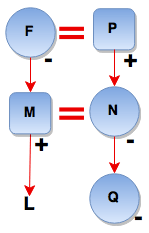# Logical Reasoning for Job Interviews - Set 3

1)   In the following question, choose the correct code form.

If R=19, RON = 50, then what FONTS equal to?

a. 75
b. 76
c. 77
d. 78
e. 79
 Answer  Explanation ANSWER: 79 Explanation: In then given series, Z = 27, Y = 26, X =25 and so on till A = 2.Then, FONTS = 7 + 16 + 15 + 21 + 20 = 79

2)   In the following question, choose the correct code form.

If BATMAN is coded as 123416, PERMAN is coded as 987416, then TAPER is coded as?

a. 32987
b. 92897
c. 38972
d. 32978

Explanation:

 Letter A B T M N P E R Code 2 1 3 4 6 9 8 7

 Letter T A P E R Code 3 2 9 8 7

3)   16 teams participated in a cricket tournament.

The tournament was conducted in two stages.

First stage
• In the first stage, the teams are divided into two equal groups.
• Each team plays every other team in its group exactly once.
• At the end of first stage, the top four teams from each group advance to the second stage.
• Rest teams are eliminated.
Second stage
• The second stage comprised several rounds.
• A round involves one match for each team. The winner of a match in a round advances to the next round, while the loser is eliminated.
• The team that remains undefeated in the second stage is declared the winner and claims the Gold Cup.
Rules
• The tournament rules such that each match results in a winner and a loser with no possibility of a tie.
• In the first stage, a team earns one point for each win and no points for a loss.
• At the end of the first stage, teams in each group are ranked on the basis of total points to determine the qualifiers advancing to the next stage.
• Ties are resolved by a series of complex tie-breaking rules so that exactly four teams from each group advanced to the next stage.
What is the minimum number of wins required for a team to guarantee advance (or possible advance) to next stage?

a. 5
b. 6
c. 7
d. 8
 Answer  Explanation ANSWER: 6 Explanation: For a team to advance to the second stage, it should be among the top 4 in its group. Total points on stake in a group is the same as the total number of matches which is 8C2 = 28.To guarantee advance, it can have 3 teams with the same or more points. There can be 5 teams with 5 points.So, 5 wins is not good enough to ensure advancement to round 2. However, 6 wins will guarantee its advance.

4)   Choose the odd pair of words

a. Volume - Litre
b. Resistance - Ohm
c. Time - Seconds
d. Length - Metre
e. Pressure - Barometer
 Answer  Explanation ANSWER: Pressure - Barometer Explanation: Barometer is an instrument.In all other pairs, second term is the unit to measure the first.

5)   Choose the odd pair.

b. Lampblack – Tyre
c. Diamond – Glass-cutter
d. Coke – Shoe polish
 Answer  Explanation ANSWER: Coke – Shoe polish Explanation: In all others, first is a necessary constituent of the second.Option D is the odd pair.

• Six cute dogs Abby, B-dawg, Charlie, Dalton, Eddie and Fantom are standing in two rows, three in each.
• Eddie is not at the end of any row.
• Dalton is second to the left of Fantom.
• Charlie, neighbor of Eddie, is standing diagonally opposite to Dalton.
• B-dawg is the neighbor of Fantom.
Who will be the neighbors of Dalton if Dalton is placed in position of Eddie?

a. Charlie and Abby
b. Fantom and B-dawg
c. Only B-dawg
d. Only Abby

Explanation:
Eddie is not at the end, so, Eddie must be in the middle of one of the rows.

Dalton is second to the left of Fantom i.e. Dalton, ___, Fantom

Charlie is the neighbor of Eddie and is standing diagonally opposite to Dalton.

B-dawg is the neighbor of Fantom.

So, the arrangement is -

 Dalton B-dawg Fantom Abby Eddie Charlie

Original neighbors of Eddie are Abby and Charlie.

So, on interchanging place with Eddie, the new neighbors of Dalton will be Abby and Charlie.

• Six cute dogs Abby, B-dawg, Charlie, Dalton, Eddie and Fantom are standing in two rows, three in each.
• Eddie is not at the end of any row.
• Dalton is second to the left of Fantom.
• Charlie, neighbor of Eddie, is standing diagonally opposite to Dalton.
• B-dawg is the neighbor of Fantom.
Which of the following are in the same row?

a. Fantom, B-dawg, Charlie
b. Charlie, Eddie, B-dawg
c. Dalton, B-dawg, Fantom
d. Abby, B-dawg, Fantom

Explanation:
Eddie is not at the end, so, Eddie must be in the middle of one of the rows.

Dalton is second to the left of Fantom i.e. Dalton, ___, Fantom

Charlie is the neighbor of Eddie and is standing diagonally opposite to Dalton.

B-dawg is the neighbor of Fantom.

So, the arrangement is –

 Dalton B-dawg Fantom Abby Eddie Charlie

Here, Dalton, B-dawg and Fantom are in the same row.

8)   In the following questions, one term in the number series is wrong. Find the wrong term.

15, 16, 20, 30, 45, 70

a. 16
b. 30
c. 45
d. 70
 Answer  Explanation ANSWER: 30 Explanation: The pattern in the given series is - +1 +4 +9 +16 +25 +36…..The wrong term is 30The correct term should be: 20 + 9 = 29

9)   In the following questions, one term in the number series is wrong. Find the wrong term.

10, 14, 28, 34, 64, 68, 136

a. 28
b. 34
c. 64
d. 68
 Answer  Explanation ANSWER: 34 Explanation: The pattern in the given series is +4 x2 +4 x2 +4 x2….The wrong number here is 34.The correct number should be: 28 + 4 = 32

10)   In the question below is given a statement followed by two courses of action numbered I and II. You have to assume everything in the statement to be true and based on the information given in the statement, decide which of the suggested courses of action logically follow(s) for pursuing.

(A) If only I follows
(B) If only II follows
(C) If either I or II follows
(D) If neither I nor II follow
(E) If both I and II follow.

Statement - Busy roads are crowded with vendors selling cheap items, leading to congestion on footpaths.

Courses of Action - I. The help of police should be sought to chase them away.

II. Some space should be provided to them where they can set up shops.

a. Only I follows
b. Only II follows
c. Either I or II follows
d. Neither I nor II follows
e. Both I and II follow
 Answer  Explanation ANSWER: Both I and II follow Explanation: Crowding on footpath creates problems for everyone, so, action should be taken to remove the vendors. At the same time, these people should be provided with alternative space without blocking the footpaths.So, both I and II follow.

11)   In the question below is given a statement followed by two courses of action numbered I and II. You have to assume everything in the statement to be true and based on the information given in the statement, decide which of the suggested courses of action logically follow(s) for pursuing.

(A) If only I follows
(B) If only II follows
(C) If either I or II follows
(D) If neither I nor II follow
(E) If both I and II follow.

Statement - As stated in the recent report the female foeticide has increased in the country.

Courses of Action - I. Government should run program to educate the masses about the importance of having female child in the society.

II. Government should immediately issue orders to all the sonography centres and hospitals to stop conducting sex tests.

a. Only I follows
b. Only II follows
c. Either I or II follows
d. Neither I nor II follows
e. Both I and II follow
 Answer  Explanation ANSWER: Both I and II follow Explanation: Female foeticide can be stopped by creating awareness among the people.And strict measures must be taken by the Government to control it. Thus, both I and II follow.

12)   In the question below is given a statement followed by two conclusions numbered I and II.

(A) If only I follows
(B) If only II follows
(C) If either I or II follows
(D) If neither I nor II follows
(E) If both I and II follow.

Conclusions - I. The assurance is genuine.

II. Girls in India want a fair skin.

a. Only I follows
b. Only II follows
c. Either I or II follows
d. Neither I nor II follows
e. Both I and II follow
 Answer  Explanation ANSWER: Only II follows Explanation: In the given statement, nothing is given about whether the advertisement is genuine or not, so, I does not follow.Since, the advertisement talks of quick fix for a fair skin, it implies that girls in India do want to get fair fast. So, II follows.

13)   In the question below is given a statement followed by two conclusions numbered I and II.

(A) If only I follows
(B) If only II follows
(C) If either I or II follows
(D) If neither I nor II follows
(E) If both I and II follow.

Statement - I know nothing except the fact of my ignorance.

Conclusions - I. The writer is a poor reader. He should read more to improve his knowledge.

II. World of knowledge is a deep ocean to be explored and conquered by a single person.

a. Only I follows
b. Only II follows
c. Either I or II follows
d. Neither I nor II follows
e. Both I and II follow
 Answer  Explanation ANSWER: Only II follows Explanation: As per the given statement, there is no way to identify whether the writer is intelligent or not. I does not follow.Here statement II correctly conveys what the given statement means.So, only II follows.

• Rambo walked 40 m towards north and killed a terrorist.
• Then he silently turned right and walked 60 m.
• Then he turned right, walks 70 m and again he turned left, walks 30 m.
• Finally, he turned left and walks 30 m.
In which direction and how far is he from the starting position?

a. 90 m East
b. 90 m West
c. 90 m South
d. 90 m North
 Answer  Explanation ANSWER: 90 m East Explanation: Required distance = AF = 60 + 30 = 90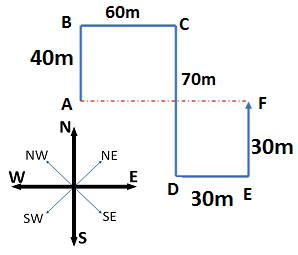15)   A sales executive went to meet his client A in another office 17 km away in the North-east direction of his own office. From there he went to see client B in an office 15 km in the south of his client A’s office. How far from the starting point and in what direction is he now?

a. 8 km, west
b. 8 km, east
c. 7 km, west
d. 7 km, east
 Answer  Explanation ANSWER: 8 km, east Explanation: The executive moves from his office O to office A and then to office B.Clearly, Triangle OAB is right-angled triangle.So, OA2 = OB2 + AB2OB2 = OA2 – AB2OB = 172 - 152 = 264 = 8Thus, the executive is 8 km to the east of his initial position.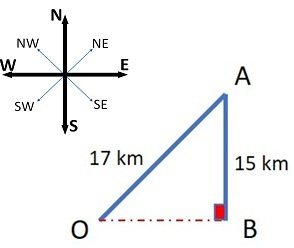1. Rohansh is the son of Aryansh’s father’s sister.
2. Somit is son of Disha who is mother of Aarav and Grandmother of Aryansh.
3. Lalit is the father of Ananya and grandfather of Rohansh.
4. Disha is wife of Lalit.

How is Disha related to Rohansh?

a. Aunt
b. Grandmother
c. Sister
d. Cousin
 Answer  Explanation ANSWER: Grandmother Explanation: Disha is wife of Lalit, and Lalit is grandfather of Rohansh.Rohansh is Disha’s grandson.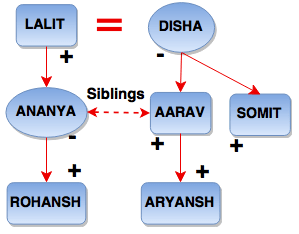1. Rohansh is the son of Aryansh’s father’s sister.
2. Somit is son of Disha who is mother of Aarav and Grandmother of Aryansh.
3. Lalit is the father of Ananya and grandfather of Rohansh.
4. Disha is wife of Lalit.

How is Aarav’s wife related to Ananya?

a. Niece
b. Mother
c. Sister
d. Sister-in-law
 Answer  Explanation ANSWER: Sister-in-law Explanation: Ananya is Lalit’s daughter, Aarav is Disha’s son and Lalit is Disha’s husband.So, Aarav is Ananya’s brother and his wife is Ananya’s sister-in-law.18)   At what time between 5.30 & 6 will the hands of a watch be at right angle?

a. 43(7/11) min past 5
b. 41(2/11) min past 5
c. 42 min past 5
d. 44(1/11) min past 5
 Answer  Explanation ANSWER: 43(7/11) min past 5 Explanation: At 5 o'clock, the hands are 25 min. spaces apart. To be at right angles and that too between 5.30 and 6, the minute hand must gain 40 min 55 min. spaces are gained in 60 min.40 min. spaces would be gained in (60/55 × 40) min. = 43 7/11 min. Required time = 43(7/11) min. past 5.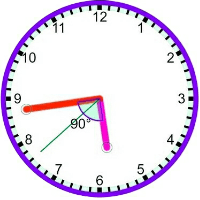19)   In the question below is given a statement followed by two conclusions numbered I and II.

(A) If only I follows
(B) If only II follows
(C) If either I or II follows
(D) If neither I nor II follows
(E) If both I and II follow.

Statement - I. No woman is stupid
II. Neha is a woman

Conclusion - I. Neha is not stupid
II. All woman are Neha

a. Only I follows
b. Only II follows
c. Either I or II follows
d. Neither I nor II follows
e. Both I and II follow
 Answer  Explanation ANSWER: Only I follows Explanation: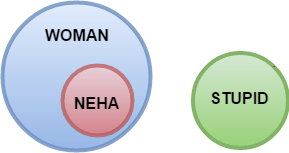1. In a family of six persons, L, M, N, F, P, Q, there are two married couples.
2. F is grandmother of L and mother of M.
3. N is wife of M and mother of Q.
4. Q is the grand-daughter of P.

What is N to L?

a. Daughter
b. Mother
c. Grandmother
d. None of these
 Answer  Explanation ANSWER: Mother Explanation: N is the wife of M and F is the mother of M.Also, F is the grandmother of L.So, N is the mother of L.